시간 제한 메모리 제한 제출 정답 맞은 사람 정답 비율
3 초 512 MB 0 0 0 0.000%

## 문제

You have a $10^9 \times 10^9$ square magnetic board with the origin of the coordinate system in the lower-left corner. There are $n$ magnets on the board, numbered from $1$ to $n$. Each magnet is an $1 \times 1$ square. Initially, the magnets are positioned in such way that the lower right corner of the $i$-th magnet has the coordinates $(i, 0)$.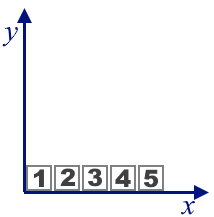Example of the initial state for $n=5$

You are receiving $q$ queries of two types:

• a query of type $1$ is characterized by two integers $l$ and $r$ ($1 \leq l \leq r \leq n$): take magnets with numbers from $l$ to $r$ inclusive, and rotate them by $90^{\circ}$. If the selected magnets formed a horizontal segment, then the rotation should be performed counterclockwise by $90^{\circ}$, so they will turn into a vertical segment. If the selected magnets formed a vertical segment, then the rotation should be performed clockwise $90^{\circ}$, so they will turn into a horizontal segment. All turns are relative to magnet with the smallest number. In this query, it is guaranteed that magnets with numbers from $l$ to $r$ form a continuous horizontal or vertical segment at the time of query processing.
• a query of type $2$ is characterized by one integer $j$ ($1 \leq j \leq n$): output the coordinates $(x, y)$ of the lower right corner of the magnet with the number $j$.

Below are the board states for $n=6$ and the series of first type queries $(l_1=2,r_1=5)$, $(l_2=3,r_2=4)$, $(l_3=2,r_3=3)$, $(l_4=6,r_4=6)$.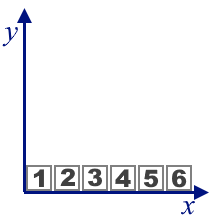Initial state for $n=6$.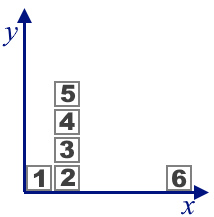After processing a query of the first type $(l_1=2,r_1=5)$.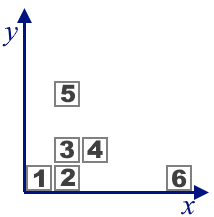After processing a query of the first type $(l_2=3,r_2=4)$.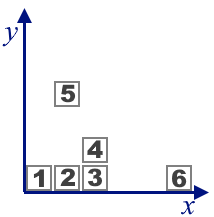After processing a query of the first type $(l_3=2,r_3=3)$.After processing a query of the first type $(l_4=6,r_4=6)$.

For each query of type $2$ you should output the coordinates of the lower right corner of the magnet with the corresponding number.

## 입력

The first line contains two integers $n$ and $q$ ($1 \le n, q \le 2 \cdot 10^5$) --- the number of magnets on the board and the number of queries, respectively.

Each of the following $q$ lines contains one query of either type $1$ or type $2$. A type $1$ query consists of $3$ integers $1$ $l$ $r$ $(1 \le l \le r \le n)$, a type $2$ query consists of $2$ integers $2$ $j$ $(1 \le j \le n)$.

## 출력

For each query of the type $2$ output $x$ and $y$ --- coordinates of the lower right corner of the magnet with the number $j$ at the moment of processing the corresponding query.

## 예제 입력 1

6 8
1 2 5
2 5
1 3 4
2 4
1 2 3
2 6
1 6 6
2 1


## 예제 출력 1

2 3
3 1
6 0
1 0


## 예제 입력 2

7 7
1 3 6
1 3 4
1 5 6
2 3
2 4
2 5
2 6


## 예제 출력 2

3 0
4 0
3 2
4 2